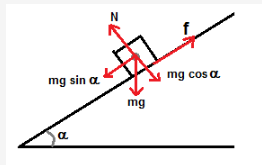# What is relation between angle of friction and angle of repose . and how we this in solving questions

what is relation between angle of friction and angle of repose . and how we this in solving questionsAngle of Friction beween any two surface in contact is defined as the angle which the resultant of the force of limiting friction Flim and normal reaction N makes with the direction of normal reaction N as shown in figure. It is marked in the figure as θ.

The value of angle of friction depends on the material and nature of surfaces in contact.
It can be seen from figure, tanθ = AC/OA = Flim / N = μ , where μ is coefficient of limiting frictionThe angle of repose or angle of sliding α is defined as the minimum angle of inclination of a plane with the horizontal such that a body placed on the plane just begins to slide down.

Its value depends on the material and nature of the surface in contact.
from the figure it can be seen that, f =mg sinα …(1)
N = mg cosα …(2)
hence we get, f / N = tan α = μ